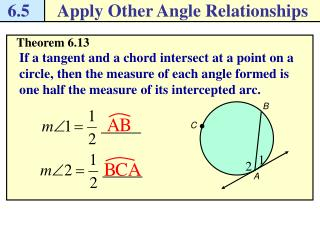DownloadDownload Presentation6.5

# 6.5

Download Presentation## 6.5

- - - - - - - - - - - - - - - - - - - - - - - - - - - E N D - - - - - - - - - - - - - - - - - - - - - - - - - - -
##### Presentation Transcript

1. 6.5 Apply Other Angle Relationships B C A Theorem 6.13 If a tangent and a chord intersect at a point on a circle, then the measure of each angle formed is one half the measure of its intercepted arc.

2. 6.5 Apply Other Angle Relationships B m E D m A F Find angle and arc measures Example 1 Line m is tangent to the circle. Find the indicated measure. Solution

3. 6.5 Apply Other Angle Relationships A B C Checkpoint. Find the indicated measure.

4. 6.5 Apply Other Angle Relationships D A C B Theorem 6.14 If two chords intersect inside a circle, then the measure of each angle is one half the sum of the measures of the arcs intercepted by the angle and its vertical angle.

5. 6.5 Apply Other Angle Relationships J F The chords FH and GJ intersect inside the circle. H G Find an angle measure inside a circle Example 2 Find the value of x. Solution

6. 6.5 Apply Other Angle Relationships F J H G Checkpoint. Find the value of x.

7. 6.5 Apply Other Angle Relationships B A X W C P Z Q Y R Theorem 6.15 If a tangent and a secant, two tangents, or two secants intersect outside a circle, then the measure of the angle formed is one half the difference of the measures of the intercepted arcs.

8. 6.5 Apply Other Angle Relationships J The tangent GF and the secant GJ intersect outside the circle. H G F Find an angle measure outside a circle Example 3 Find the value of x. Solution

9. 6.5 Apply Other Angle Relationships G H J F K Checkpoint. Find the value of y.

10. 6.5 Apply Other Angle Relationships Pg. 225, 6.5 #1-23